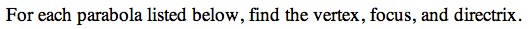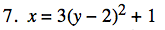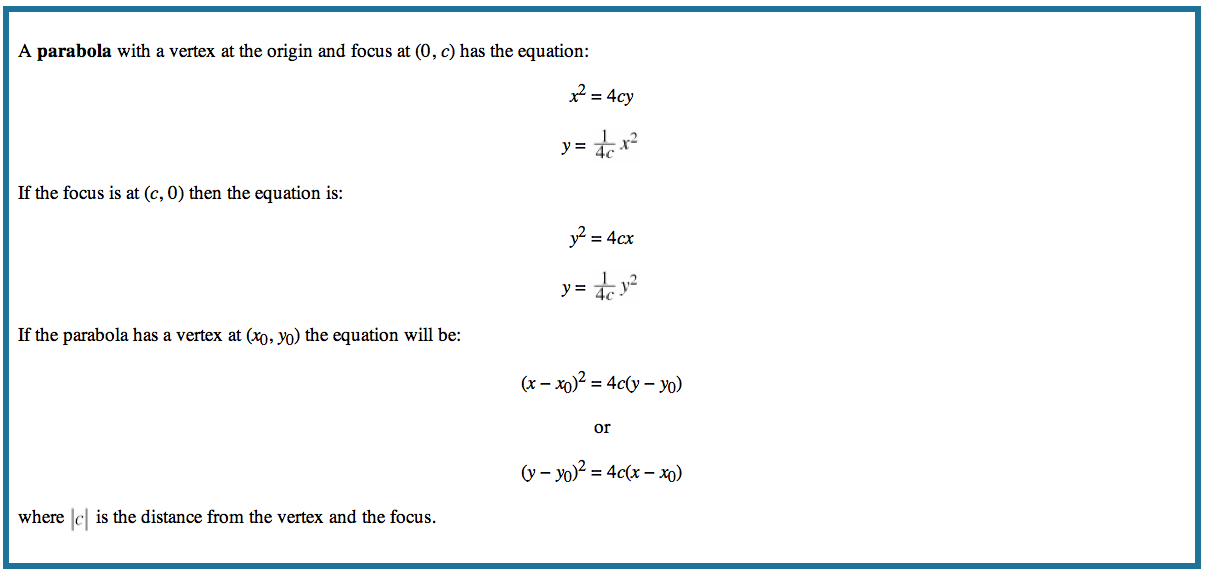13P-7.

### Missing problem for Book PCT => Chapter Ch13 => Lesson 13.1.4 => Problem 13P-7

Created from orphaned homework help problemSubtract 1 from both sides, then divide the equation by 3.

$\frac{1}{3}\left(\textit{x}-1\right)=\left(\textit{y}-2\right)^2$